# Tag: Borcherds

An integral $n$-dimensional lattice $L$ is the set of all integral linear combinations
$L = \mathbb{Z} \lambda_1 \oplus \dots \oplus \mathbb{Z} \lambda_n$
of base vectors $\{ \lambda_1,\dots,\lambda_n \}$ of $\mathbb{R}^n$, equipped with the usual (positive definite) inner product, satisfying
$(\lambda, \mu ) \in \mathbb{Z} \quad \text{for all \lambda,\mu \in \mathbb{Z}.}$
But then, $L$ is contained in its dual lattice $L^* = Hom_{\mathbb{Z}}(L,\mathbb{Z})$, and if $L = L^*$ we say that $L$ is unimodular.

If all $(\lambda,\lambda) \in 2 \mathbb{Z}$, we say that $L$ is an even lattice. Even unimodular lattices (such as the $E_8$-lattice or the $24$ Niemeier lattices) are wonderful objects, but they can only live in dimensions $n$ which are multiples of $8$.

Just like the Conway group $Co_0 = .0$ is the group of rotations of the Leech lattice $\Lambda$, one might ask whether there is a very special lattice on which the Monster group $\mathbb{M}$ acts faithfully by rotations. If such a lattice exists, it must live in dimension at least $196883$.Simon Norton (1952-2019) – Photo Credit

A first hint of such a lattice is in Conway’s original paper A simple construction for the Fischer-Griess monster group (but not in the corresponding chapter 29 of SPLAG).

Conway writes that Simon Norton showed ‘by a very simple computations that does not even require knowledge of the conjugacy classes, that any $198883$-dimensional representation of the Monster must support an invariant algebra’, which, after adding an identity element $1$, we now know as the $196884$-dimensional Griess algebra.

Further, on page 529, Conway writes:

Norton has shown that the lattice $L$ spanned by vectors of the form $1,t,t \ast t’$, where $t$ and $t’$ are transposition vectors, is closed under the algebra multiplication and integral with respect to the doubled inner product $2(u,v)$. The dual quotient $L^*/L$ is cyclic of order some power of $4$, and we believe that in fact $L$ is unimodular.

Here, transposition vectors correspond to transpositions in $\mathbb{M}$, that is, elements of conjugacy class $2A$.

In his post, Adam considers the $196883$-dimensional lattice $L’ = L \cap 1^{\perp}$ (which has $\mathbb{M}$ as its rotation symmetry group), and asks for the minimal norm (squared) of a lattice point, which he believes is $448$, and for the number of minimal vectors in the lattice, which might be
$2639459181687194563957260000000 = 9723946114200918600 \times 27143910000$
the number of oriented arcs in the Monster graph.

Here, the Monster graph has as its vertices the elements of $\mathbb{M}$ in conjugacy class $2A$ (which has $9723946114200918600$ elements) and with an edge between two vertices if their product in $\mathbb{M}$ again belongs to class $2A$, so the valency of the graph must be $27143910000$, as explained in that old post the monster graph and McKay’s observation.

When I asked Adam whether he had more information about his lattice, he kindly informed me that Borcherds told him that the Norton lattice $L$ didn’t turn out to be unimodular after all, but that a unimodular lattice with monstrous symmetry had been constructed by Scott Carnahan in the paper A Self-Dual Integral Form of the Moonshine Module.Scott Carnahan – Photo Credit

The major steps (or better, the little bit of it I could grasp in this short time) in the construction of this unimodular $196884$-dimensional monstrous lattice might put a smile on your face if you are an affine scheme aficionado.

Already in his paper Vertex algebras, Kac-Moody algebras, and the Monster, Richard Borcherds described an integral form of any lattice vertex algebra. We’ll be interested in the lattice vertex algebra $V_{\Lambda}$ constructed from the Leech lattice $\Lambda$ and call its integral form $(V_{\Lambda})_{\mathbb{Z}}$.

One constructs the Moonshine module $V^{\sharp}$ from $V_{\Lambda}$ by a process called ‘cyclic orbifolding’, a generalisation of the original construction by Frenkel, Lepowsky and Meurman. In fact, there are now no less than 51 constructions of the moonshine module.

One starts with a fixed point free rotation $r_p$ of $\Lambda$ in $Co_0$ of prime order $p \in \{ 2,3,5,7,13 \}$, which one can lift to an automorphism $g_p$ of the vertex algebra $V_{\Lambda}$ of order $p$ giving an isomorphism $V_{\Lambda}/g_p \simeq V^{\sharp}$ of vertex operator algebras over $\mathbb{C}$.

For two distinct primes $p,p’ \in \{ 2,3,5,7,13 \}$ if $Co_0$ has an element of order $p.p’$ one can find one such $r_{pp’}$ such that $r_{pp’}^p=r_{p’}$ and $r_{pp’}^{p’}=r_p$, and one can lift $r_{pp’}$ to an automorphism $g_{pp’}$ of $V_{\Lambda}$ such that $V_{\Lambda}/g_{pp’} \simeq V_{\Lambda}$ as vertex operator algebras over $\mathbb{C}$.

Problem is that these lifts of automorphisms and the isomorphisms are not compatible with the integral form $(V_{\Lambda})_{\mathbb{Z}}$ of $V_{\Lambda}$, but ‘essentially’, they can be performed on
$(V_{\Lambda})_{\mathbb{Z}} \otimes_{\mathbb{Z}} \mathbb{Z}[\frac{1}{pp’},\zeta_{2pp’}]$
where $\zeta_{2pp’}$ is a primitive $2pp’$-th root of unity. These then give a $\mathbb{Z}[\tfrac{1}{pp’},\zeta_{2pp’}]$-form on $V^{\sharp}$.

Next, one uses a lot of subgroup information about $\mathbb{M}$ to prove that these $\mathbb{Z}[\tfrac{1}{pp’},\zeta_{2pp’}]$-forms of $V^{\sharp}$ have $\mathbb{M}$ as their automorphism group.

Then, using all his for different triples in $\{ 2,3,5,7,13 \}$ one can glue and use faithfully flat descent to get an integral form $V^{\sharp}_{\mathbb{Z}}$ of the moonshine module with monstrous symmetry and such that the inner product on $V^{\sharp}_{\mathbb{Z}}$ is positive definite.

Finally, one looks at the weight $2$ subspace of $V^{\sharp}_{\mathbb{Z}}$ which gives us our Carnahan’s $196884$-dimensional unimodular lattice with monstrous symmetry!

Beautiful as this is, I guess it will be a heck of a project to deduce even the simplest of facts about this wonderful lattice from running through this construction.

For example, what is the minimal length of vectors? What is the number of minimal length vectors? And so on. All info you might have is very welcome.

No, this is not another timely post about the British Royal family.

It’s about Richard Borcherds’ “teapot test” for quantum computers.A lot of money is being thrown at the quantum computing hype, causing people to leave academia for quantum computing firms. A recent example (hitting the press even in Belgium) being the move of Bob Coecke from Oxford University to Cambridge Quantum Computing.

Sure, quantum computing is an enticing idea, and we have fantastic quantum algorithms such as Shor’s factorisation algorithm and Grover’s search algorithm.

The (engineering) problem is building quantum computers with a large enough number of qubits, which is very difficult due to quantum decoherence. To an outsider it may appear that the number of qubits in a working quantum computer is growing at best linearly, if not logarithmic, in sharp contrast to Moore’s law for classical computers, stating that the number of transistors in an integrated circuit doubles every two years.

Quantum computing evangelists assure us that this is nonsense, and that we should replace Moore’s law by Neven’s law claiming that the computing power of quantum computers will grow not just exponentially, but doubly exponentially!

What is behind these exaggerated claims?

In 2015 the NSA released a policy statement on the need for post-quantum cryptography. In the paper “A riddle wrapped in an enigma”, Neil Koblitz and Alfred Menezes carefully examined NSA’s possible strategies behind this assertion.

Can the NSA break PQC? Can the NSA break RSA? Does the NSA believes that RSA-3072 is much more quantum-resistant than ECC-256 and even ECC-384?, and so on.

Perhaps the most plausible of all explanations is this one : the NSA is using a diversion strategy aimed at Russia and China.

Suppose that the NSA believes that, although a large-scale quantum computer might eventually be built, it will be hugely expensive. From a cost standpoint it will be less analogous to Alan Turing’s bombe than to the Manhattan Project or the Apollo program, and it will be within the capabilities of only a small number of nation-states and huge corporations.

Suppose also that, in thinking about the somewhat adversarial relationship that still exists between the U.S. and both China and Russia, especially in the area of cybersecurity, the NSA asked itself “How did we win the Cold War? The main strategy was to goad the Soviet Union into an arms race that it could not afford, essentially bankrupting it. Their GNP was so much less than ours, what was a minor set-back for our economy was a major disaster for theirs. It was a great strategy. Let’s try it again.”

This brings us to the claim of quantum supremacy, that is, demonstrating that a programmable quantum device can solve a problem that no classical computer can solve in any feasible amount of time.

In 2019, Google claimed “to have reached quantum supremacy with an array of 54 qubits out of which 53 were functional, which were used to perform a series of operations in 200 seconds that would take a supercomputer about 10,000 years to complete”. In December 2020, a group based in USTC reached quantum supremacy by implementing a type of Boson sampling on 76 photons with their photonic quantum computer. They stated that to generate the number of samples the quantum computer generates in 20 seconds, a classical supercomputer would require 600 million years of computation.

Richard Borcherds rants against the type of problems used to claim quantum ‘supremacy’. He proposes the ‘teapot problem’ which a teapot can solve instantaneously, but will be impossibly hard for classical (and even quantum) computers. That is, any teapot achieves ‘teapot supremacy’ over classical and quantum computers!

Another point of contention are the ‘real-life applications’ quantum computers are said to be used for. Probably he is referring to Volkswagen’s plan for traffic optimization with a D-Wave quantum computer in Lisbon.

“You could give these guys a time machine and all they’d use it for was going back to watch some episodes of some soap opera they missed”

Enjoy!

Whenever I visit someone’s YouTube or Twitter profile page, I hope to see an interesting banner image. Here’s the one from Richard Borcherds’ YouTube Channel.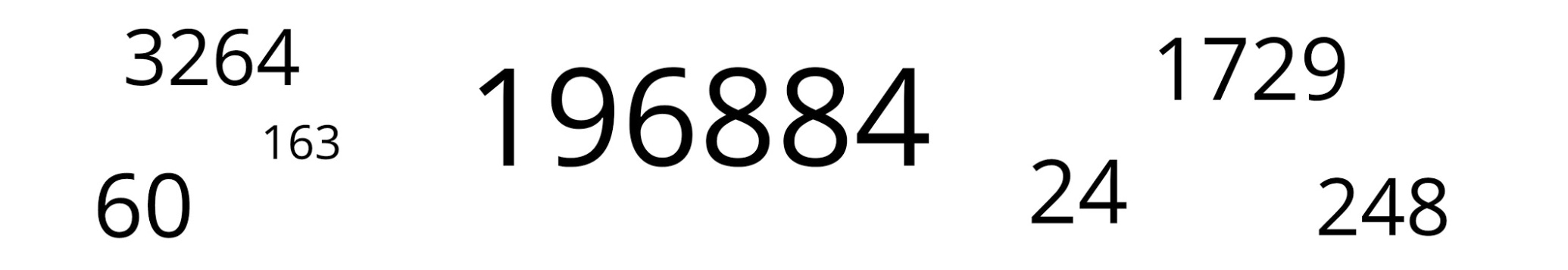Not too surprisingly for Borcherds, almost all of these numbers are related to the monster group or its moonshine.

Let’s try to decode them, in no particular order.

196884

John McKay’s observation $196884 = 1 + 196883$ was the start of the whole ‘monstrous moonshine’ industry. Here, $1$ and $196883$ are the dimensions of the two smallest irreducible representations of the monster simple group, and $196884$ is the first non-trivial coefficient in Klein’s j-function in number theory.

$196884$ is also the dimension of the space in which Robert Griess constructed the Monster, following Simon Norton’s lead that there should be an algebra structure on the monster-representation of that dimension. This algebra is now known as the Griess algebra.

Here’s a recent talk by Griess “My life and times with the sporadic simple groups” in which he tells about his construction of the monster (relevant part starting at 1:15:53 into the movie).

1729

1729 is the second (and most famous) taxicab number. A long time ago I did write a post about the classic Ramanujan-Hardy story the taxicab curve (note to self: try to tidy up the layout of some old posts!).

Recently, connections between Ramanujan’s observation and K3-surfaces were discovered. Emory University has an enticing press release about this: Mathematicians find ‘magic key’ to drive Ramanujan’s taxi-cab number. The paper itself is here.

“We’ve found that Ramanujan actually discovered a K3 surface more than 30 years before others started studying K3 surfaces and they were even named. It turns out that Ramanujan’s work anticipated deep structures that have become fundamental objects in arithmetic geometry, number theory and physics.”

Ken Ono

24

There’s no other number like $24$ responsible for the existence of sporadic simple groups.

24 is the length of the binary Golay code, with isomorphism group the sporadic Mathieu group $M_24$ and hence all of the other Mathieu-groups as subgroups.

24 is the dimension of the Leech lattice, with isomorphism group the Conway group $Co_0 = .0$ (dotto), giving us modulo its center the sporadic group $Co_1=.1$ and the other Conway groups $Co_2=.2, Co_3=.3$, and all other sporadics of the second generation in the happy family as subquotients (McL,HS,Suz and $HJ=J_2$)24 is the central charge of the Monster vertex algebra constructed by Frenkel, Lepowski and Meurman. Most experts believe that the Monster’s reason of existence is that it is the symmetry group of this vertex algebra. John Conway was one among few others hoping for a nicer explanation, as he said in this interview with Alex Ryba.

24 is also an important number in monstrous moonshine, see for example the post the defining property of 24. There’s a lot more to say on this, but I’ll save it for another day.

60

60 is, of course, the order of the smallest non-Abelian simple group, $A_5$, the rotation symmetry group of the icosahedron. $A_5$ is the symmetry group of choice for most viruses but not the Corona-virus.

3264

3264 is the correct solution to Steiner’s conic problem asking for the number of conics in $\mathbb{P}^2_{\mathbb{C}}$ tangent to five given conics in general position.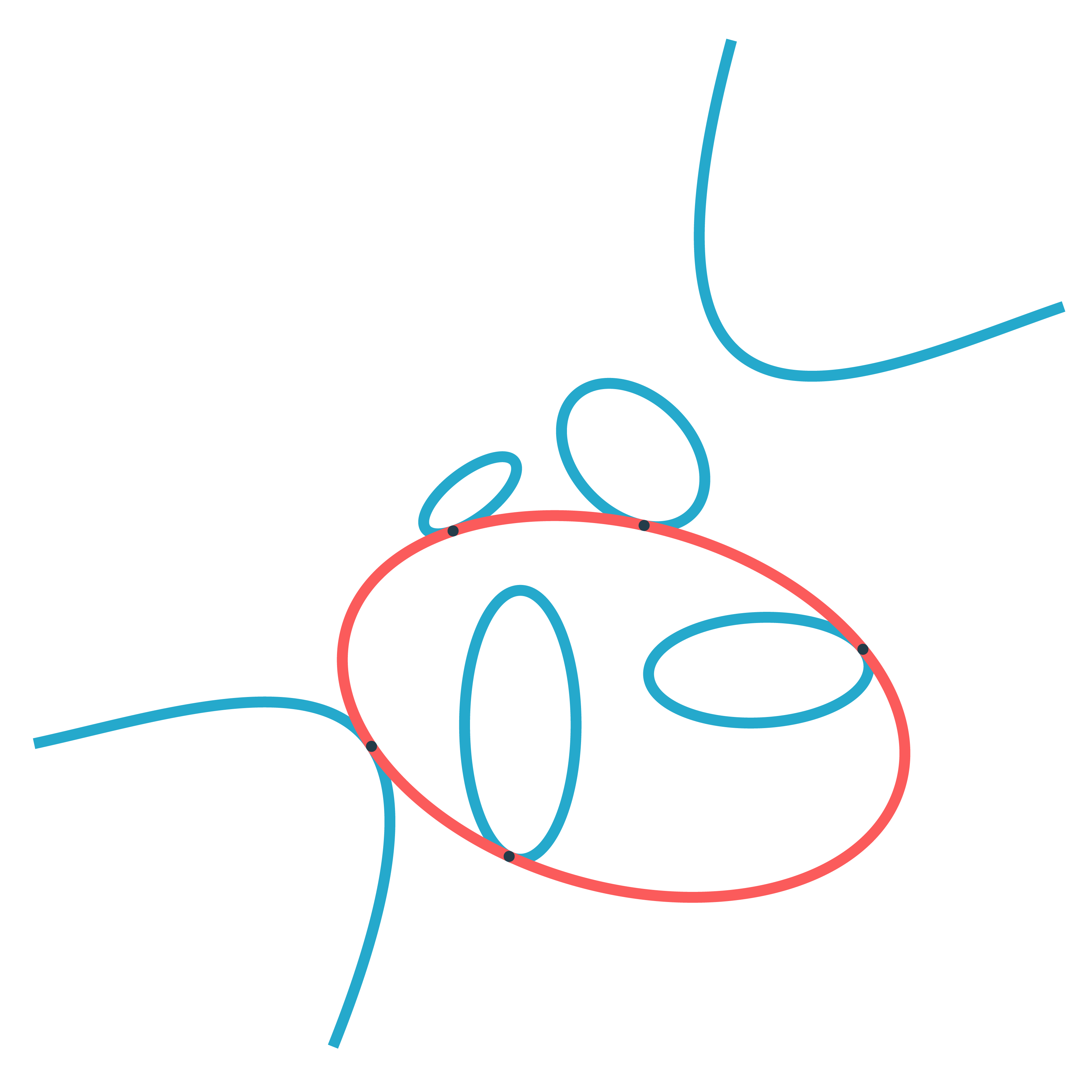Steiner himself claimed that there were $7776=6^5$ such conics, but realised later that he was wrong. The correct number was first given by Ernest de Jonquières in 1859, but a rigorous proof had to await the advent of modern intersection theory.

Eisenbud and Harris wrote a book on intersection theory in algebraic geometry, freely available online: 3264 and all that.

248

248 is the dimension of the exceptional simple Lie group $E_8$. $E_8$ is also connected to the monster group.

If you take two Fischer involutions in the monster (elements of conjugacy class 2A) and multiply them, the resulting element surprisingly belongs to one of just 9 conjugacy classes:

1A,2A,2B,3A,3C,4A,4B,5A or 6A

The orders of these elements are exactly the dimensions of the fundamental root for the extended $E_8$ Dynkin diagram.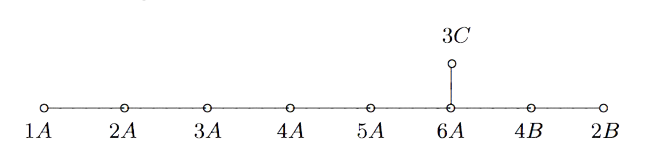This is yet another moonshine observation by John McKay and I wrote a couple of posts about it and about Duncan’s solution: the monster graph and McKay’s observation, and $E_8$ from moonshine groups.

163

163 is a remarkable number because of the ‘modular miracle’
$e^{\pi \sqrt{163}} = 262537412640768743.99999999999925…$
This is somewhat related to moonshine, or at least to Klein’s j-function, which by a result of Kronecker’s detects the classnumber of imaginary quadratic fields $\mathbb{Q}(\sqrt{-D})$ and produces integers if the classnumber is one (as is the case for $\mathbb{Q}(\sqrt{-163})$).

The details are in the post the miracle of 163, or in the paper by John Stillwell, Modular Miracles, The American Mathematical Monthly, 108 (2001) 70-76.

His description of the $j$-function (at 4:13 in the movie) is simply hilarious!

Borcherds connects $163$ to the monster moonshine via the $j$-function, but there’s another one.

The monster group has $194$ conjugacy classes and monstrous moonshine assigns a ‘moonshine function’ to each conjugacy class (the $j$-function is assigned to the identity element). However, these $194$ functions are not linearly independent and the space spanned by them has dimension exactly $163$.

A rare benefit of the Covid-situation is that Richard Borcherds decided to set up a YouTube channel with recordings of his online lectures.

Plenty of gems to be discovered there, including a talk on Monstrous Moonshine, and a talk he gave for the Archimedeans about the Sporadic Groups.

As part of his History of Science-course he addressed the question whether Witt discovered the Leech lattice.

A while ago I’ve blogged about that very same question here:

The summary of these posts being that I thought it was rather unlikely for Witt to have discovered the Leech lattice.

However, using the same sources, Borcherds rates a more than 90% probability for Witt to have indeed discovered the Leech lattice in 1940.

His evidence for this is:

• Witt said he discovered it.
• His construction (in his 1972 colloquium talk) is unlike any other construction of the Leech lattice.
• Witt was the expert on Steiner systems, and the system S(5,8,24) is crucial in Leech’s construction of his lattice.

Do we know why the monster exists and why there’s moonshine around it?

The answer depends on whether or not you believe that vertex operator algebras are natural, elegant and inescapable objects.

the monster

Simple groups often arise from symmetries of exceptionally nice mathematical objects.

The smallest of them all, $A_5$, gives us the rotation symmetries of the icosahedron. The next one, Klein’s simple group $L_2(7)$, comes from the Klein quartic.

The smallest sporadic groups, the Mathieu groups, come from Steiner systems, and the Conway groups from the 24-dimensional Leech lattice.

What about the largest sporadic simple, the monster $\mathbb{M}$?

In his paper What is … the monster? Richard Borcherds writes (among other characterisations of $\mathbb{M}$):

“3. It is the automorphism group of the monster vertex algebra. (This is probably the best answer.)”

“Unfortunately none of these definitions is completely satisfactory. At the moment all constructions of the algebraic structures above seem artificial; they are constructed as sums of two or more apparently unrelated spaces, and it takes a lot of effort to define the algebraic structure on the sum of these spaces and to check that the monster acts on the resulting structure.
It is still an open problem to find a really simple and natural construction of the monster vertex algebra.

Here’s 2 minutes of John Conway on the “one thing” he really wants to know before he dies: why the monster group exists.

moonshine

Moonshine started off with McKay’s observation that 196884 (the first coefficient in the normalized j-function) is the sum 1+196883 of the dimensions of the two smallest simple representations of $\mathbb{M}$.

Soon it was realised that every conjugacy class of the monster has a genus zero group (or ‘moonshine group’) associated to it.

Borcherds proved the ‘monstrous moonshine conjectures’ asserting that the associated main modular function of such a group is the character series of the action of the element on the monster vertex algebra.

Here’s Borcherds’ ICM talk in Berlin on this: What is … Moonshine?.

Once again, the monster vertex algebra appears to be the final answer.

However, in characterising the 171 moonshine groups among all possible genus zero groups one has proved that they are all of the form:

(ii) : $(n|h)+e,g,\dots$

In his book Moonshine beyond the Monster, Terry Gannon writes:

“We now understand the significance, in the VOA or CFT framework, of transformations in $SL_2(\mathbb{Z})$, but (ii) emphasises that many modular transformations relevant to Moonshine are more general (called the Atkin-Lehner involutions).
Monstrous moonshine will remain mysterious until we can understand its Atkin-Lehner symmetries.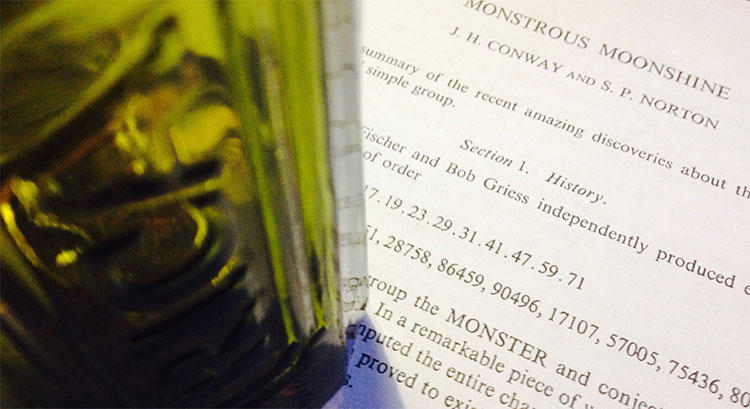At a seminar at the College de France in 1975, Tits wrote down the order of the monster group

$\# \mathbb{M} = 2^{46}.3^{20}.5^9.7^6.11^2.13^3.17·19·23·29·31·41·47·59·71$

Andrew Ogg, who attended the talk, noticed that the prime divisors are precisely the primes $p$ for which the characteristic $p$ super-singular $j$-invariants are all defined over $\mathbb{F}_p$.

Here’s Ogg’s paper on this: Automorphismes de courbes modulaires, Séminaire Delange-Pisot-Poitou. Théorie des nombres, tome 16, no 1 (1974-1975).

Ogg offered a bottle of Jack Daniels for an explanation of this coincidence.

Even Richard Borcherds didn’t claim the bottle of Jack Daniels, though his proof of the monstrous moonshine conjecture is believed to be the best explanation, at present.

A few years ago, John Duncan and Ken Ono posted a paper “The Jack Daniels Problem”, in which they prove that monstrous moonshine implies that if $p$ is not one of Ogg’s primes it cannot be a divisor of $\# \mathbb{M}$. However, the other implication remains mysterious.

Duncan and Ono say:

“This discussion does not prove that every $p ∈ \text{Ogg}$ divides $\# \mathbb{M}$. It merely explains how the first principles of moonshine suggest this implication. Monstrous moonshine is the proof. Does this then provide a completely satisfactory solution to Ogg’s problem? Maybe or maybe not. Perhaps someone will one day furnish a map from the characteristic $p$ supersingular $j$-invariants to elements of order $p$ where the group structure of $\mathbb{M}$ is apparent.”

I don’t know whether they claimed the bottle, anyway.

But then, what is the non-commutative Jack Daniels Problem?

A footnote on the first page of Conway and Norton’s ‘Monstrous Moonshine’ paper says:

“Very recently, A. Pizer has shown these primes are the only ones that satisfy a certain conjecture of Hecke from 1936 relating modular forms of weight $2$ to quaternion algebra theta-series.”

Pizer’s paper is “A note on a conjecture of Hecke”.

Maybe there’s a connection between monstrous moonshine and the arithmetic of integral quaternion algebras. Some hints:

The commutation relations in the Big Picture are reminiscent of the meta-commutation relations for Hurwitz quaternions, originally due to Conway in his booklet on Quaternions and Octonions.

The fact that the $p$-tree in the Big Picture has valency $p+1$ comes from the fact that the Brauer-Severi of $M_2(\mathbb{F}_p)$ is $\mathbb{P}^1_{\mathbb{F}_p}$. In fact, the Big Picture should be related to the Brauer-Severi scheme of $M_2(\mathbb{Z})$.

Then, there’s Jorge Plazas claiming that Connes-Marcolli’s $GL_2$-system might be related to moonshine.

One of the first things I’ll do when I return is to run to the library and get our copy of Shimura’s ‘Introduction to the arithmetic theory of automorphic functions’.

Btw. the bottle in the title image is not a Jack Daniels but the remains of a bottle of Ricard, because I’m still in the French mountains.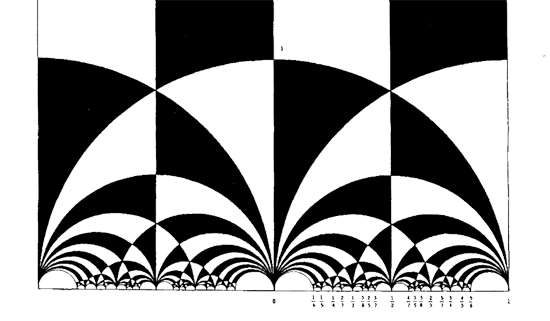The black&white psychedelic picture on the left of a tessellation of the hyperbolic upper-halfplane, was called the Dedekind tessellation in this post, following the reference given by John Stillwell in his excellent paper Modular Miracles, The American Mathematical Monthly, 108 (2001) 70-76.

But is this correct terminology? Nobody else uses it apparently. So, let’s try to track down the earliest depiction of this tessellation in the literature…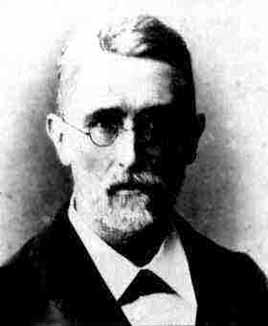Stillwell refers to Richard Dedekind‘s 1877 paper “Schreiben an Herrn Borchard uber die Theorie der elliptische Modulfunktionen”, which appeared beginning of september 1877 in Crelle’s journal (Journal fur die reine und angewandte Mathematik, Bd. 83, 265-292).

There are a few odd things about this paper. To start, it really is the transcript of a (lengthy) letter to Herrn Borchardt (at first, I misread the recipient as Herrn Borcherds which would be really weird…), written on June 12th 1877, just 2 and a half months before it appeared… Even today in the age of camera-ready-copy it would probably take longer.

There isn’t a single figure in the paper, but, it is almost impossible to follow Dedekind’s arguments without having a mental image of the tessellation. He gives a fundamental domain for the action of the modular group $\Gamma = PSL_2(\mathbb{Z})$ on the hyperbolic upper-half plane (a fact already known to Gauss) and goes on in section 3 to give a one-to-one mapping between this domain and the complex plane using what he calls the ‘valenz’ function $v$ (which is our modular function $j$, making an appearance in moonshine, and responsible for the black&white tessellation, the two colours corresponding to pre-images of the upper or lower half-planes).

Then there is this remarkable opening sentence.

Sie haben mich aufgefordert, eine etwas ausfuhrlichere Darstellung der Untersuchungen auszuarbeiten, von welchen ich, durch das Erscheinen der Abhandlung von Fuchs veranlasst, mir neulich erlaubt habe Ihnen eine kurze Ubersicht mitzuteilen; indem ich Ihrer Einladung hiermit Folge leiste, beschranke ich mich im wesentlichen auf den Teil dieser Untersuchungen, welcher mit der eben genannten Abhandlung zusammenhangt, und ich bitte Sie auch, die Ubergehung einiger Nebenpunkte entschuldigen zu wollen, da es mir im Augenblick an Zeit fehlt, alle Einzelheiten auszufuhren.

Well, just try to get a paper (let alone a letter) accepted by Crelle’s Journal with an opening line like : “I’ll restrict to just a few of the things I know, and even then, I cannot be bothered to fill in details as I don’t have the time to do so right now!” But somehow, Dedekind got away with it.

So, who was this guy Borchardt? How could this paper be published so swiftly? And, what might explain this extreme ‘je m’en fous’-opening ?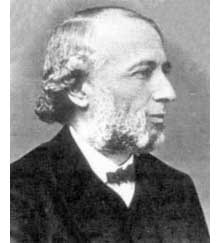Carl Borchardt was a Berlin mathematician whose main claim to fame seems to be that he succeeded Crelle in 1856 as main editor of the ‘Journal fur reine und…’ until 1880 (so in 1877 he was still in charge, explaining the swift publication). It seems that during this time the ‘Journal’ was often referred to as “Borchardt’s Journal” or in France as “Journal de M Borchardt”. After Borchardt’s death, the Journal für die Reine und Angewandte Mathematik again became known as Crelle’s Journal.

As to the opening sentence, I have a toy-theory of what was going on. In 1877 a bitter dispute was raging between Kronecker (an editor for the Journal and an important one as he was the one succeeding Borchardt when he died in 1880) and Cantor. Cantor had published most of his papers at Crelle and submitted his latest find : there is a one-to-one correspondence between points in the unit interval [0,1] and points of d-dimensional space! Kronecker did everything in his power to stop that paper to the extend that Cantor wanted to retract it and submit it elsewhere. Dedekind supported Cantor and convinced him not to retract the paper and used his influence to have the paper published in Crelle in 1878. Cantor greatly resented Kronecker’s opposition to his work and never submitted any further papers to Crelle’s Journal.

Clearly, Borchardt was involved in the dispute and it is plausible that he ‘invited’ Dedekind to submit a paper on his old results in the process. As a further peace offering, Dedekind included a few ‘nice’ words for Kronecker

Bei meiner Versuchen, tiefer in diese mir unentbehrliche Theorie einzudringen und mir einen einfachen Weg zu den ausgezeichnet schonen Resultaten von Kronecker zu bahnen, die leider noch immer so schwer zuganglich sind, enkannte ich sogleich…

Probably, Dedekind was referring to Kronecker’s relation between class groups of quadratic imaginary fields and the j-function, see the miracle of 163. As an added bonus, Dedekind was elected to the Berlin academy in 1880…

Anyhow, no visible sign of ‘Dedekind’s’ tessellation in the 1877 Dedekind paper, so, we have to look further. I’m fairly certain to have found the earliest depiction of the black&white tessellation (if you have better info, please drop a line). Here it is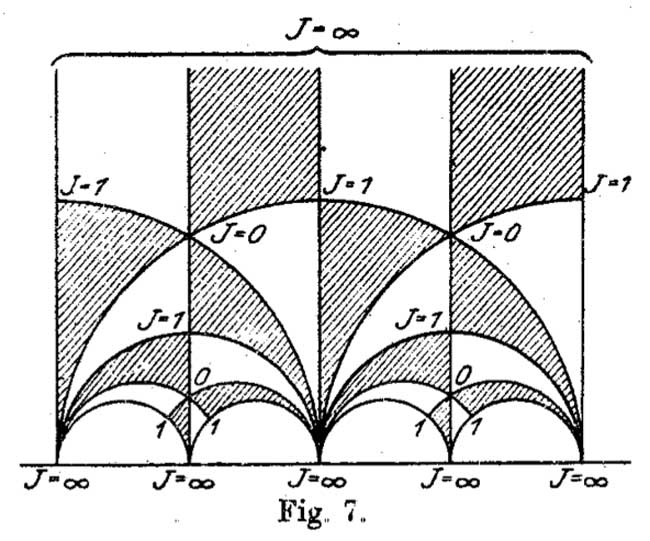It is figure 7 in Felix Klein‘s paper “Uber die Transformation der elliptischen Funktionen und die Auflosung der Gleichungen funften Grades” which appeared in may 1878 in the Mathematische Annalen (Bd. 14 1878/79). He even adds the j-values which make it clear why black triangles should be oriented counter-clockwise and white triangles clockwise. If Klein would still be around today, I’m certain he’d be a metapost-guru.

So, perhaps the tessellation should be called Klein’s tessellation??
Well, not quite. Here’s what Klein writes wrt. figure 7

Diese Figur nun – welche die eigentliche Grundlage fur das Nachfolgende abgibt – ist eben diejenige, von der Dedekind bei seiner Darstellung ausgeht. Er kommt zu ihr durch rein arithmetische Betrachtung.

Case closed : Klein clearly acknowledges that Dedekind did have this picture in mind when writing his 1877 paper!

But then, there are a few odd things about Klein’s paper too, and, I do have a toy-theory about this as well… (tbc)

Monstrous moonshine was born (sometime in 1978) the moment John McKay realized that the linear term in the j-function

$j(q) = \frac{1}{q} + 744 + 196884 q + 21493760 q^2 + 864229970 q^3 + \ldots$

is surprisingly close to the dimension of the smallest non-trivial irreducible representation of the monster group, which is 196883. Note that at that time, the Monster hasn’t been constructed yet, and, the only traces of its possible existence were kept as semi-secret information in a huge ledger (costing 80 pounds…) kept in the Atlas-office at Cambridge. Included were 8 huge pages describing the character table of the monster, the top left fragment, describing the lower dimensional irreducibles and their characters at small order elements, reproduced below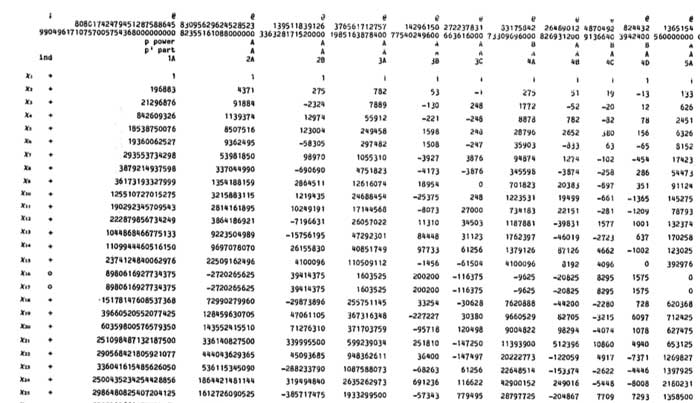If you look at the dimensions of the smallest irreducible representations (the first column) : 196883, 21296876, 842609326, … you will see that the first, second and third of them are extremely close to the linear, quadratic and cubic coefficient of the j-function. In fact, more is true : one can obtain these actual j-coefficients as simple linear combination of the dimensions of the irrducibles :

$\begin{cases} 196884 &= 1 + 196883 \\ 21493760 &= 1 + 196883 + 21296876 \\ 864229970 &= 2 \times 1 + 2 \times 196883 + 21296876 + 842609326 \end{cases}$

Often, only the first relation is attributed to McKay, whereas the second and third were supposedly discovered by John Thompson after MKay showed him the first. Marcus du Sautoy tells a somewhat different sory in Finding Moonshine :

McKay has also gone on to find these extra equations, but is was Thompson who first published them. McKay admits that “I was a bit peeved really, I don’t think Thompson quite knew how much I knew.”

By the work of Richard Borcherds we now know the (partial according to some) explanation behind these numerical facts : there is a graded representation $V = \oplus_i V_i$ of the Monster-group (actually, it has a lot of extra structure such as being a vertex algebra) such that the dimension of the i-th factor $V_i$ equals the coefficient f $q^i$ in the j-function. The homogeneous components $V_i$ being finite dimensional representations of the monster, they decompose into the 194 irreducibles $X_j$. For the first three components we have the decompositions

$\begin{cases} V_1 &= X_1 \oplus X_2 \\ V_2 &= X_1 \oplus X_2 \oplus X_3 \\ V_3 &= X_1^{\oplus 2 } \oplus X_2^{\oplus 2} \oplus X_3 \oplus X_4 \end{cases}$

Calculating the dimensions on both sides give the above equations. However, being isomorphisms of monster-representations we are not restricted to just computing the dimensions. We might as well compute the character of any monster-element on both sides (observe that the dimension is just the character of the identity element). Characters are the traces of the matrices describing the action of a monster-element on the representation and these numbers fill the different columns of the character-table above.

Hence, the same integral combinations of the character values of any monster-element give another q-series and these are called the McKay-Thompson series. John Conway discovered them to be classical modular functions known as Hauptmoduln.

In most papers and online material on this only the first few coefficients of these series are documented, which may be just too little information to make new discoveries!

Fortunately, David Madore has compiled the first 3200 coefficients of all the 172 monster-series which are available in a huge 8Mb file. And, if you really need to have more coefficients, you can always use and modify his moonshine python program.

In order to reduce bandwidth, here a list containing the first 100 coefficients of the j-function

jfunct=[196884, 21493760, 864299970, 20245856256, 333202640600, 4252023300096, 44656994071935, 401490886656000, 3176440229784420, 22567393309593600, 146211911499519294, 874313719685775360, 4872010111798142520, 25497827389410525184, 126142916465781843075, 593121772421445058560, 2662842413150775245160, 11459912788444786513920, 47438786801234168813250, 189449976248893390028800, 731811377318137519245696, 2740630712513624654929920, 9971041659937182693533820, 35307453186561427099877376, 121883284330422510433351500, 410789960190307909157638144, 1353563541518646878675077500, 4365689224858876634610401280, 13798375834642999925542288376, 42780782244213262567058227200, 130233693825770295128044873221, 389608006170995911894300098560, 1146329398900810637779611090240, 3319627709139267167263679606784, 9468166135702260431646263438600, 26614365825753796268872151875584, 73773169969725069760801792854360, 201768789947228738648580043776000, 544763881751616630123165410477688, 1452689254439362169794355429376000, 3827767751739363485065598331130120, 9970416600217443268739409968824320, 25683334706395406994774011866319670, 65452367731499268312170283695144960, 165078821568186174782496283155142200, 412189630805216773489544457234333696, 1019253515891576791938652011091437835, 2496774105950716692603315123199672320, 6060574415413720999542378222812650932, 14581598453215019997540391326153984000, 34782974253512490652111111930326416268, 82282309236048637946346570669250805760, 193075525467822574167329529658775261720, 449497224123337477155078537760754122752, 1038483010587949794068925153685932435825, 2381407585309922413499951812839633584128, 5421449889876564723000378957979772088000, 12255365475040820661535516233050165760000, 27513411092859486460692553086168714659374, 61354289505303613617069338272284858777600, 135925092428365503809701809166616289474168, 299210983800076883665074958854523331870720, 654553043491650303064385476041569995365270, 1423197635972716062310802114654243653681152, 3076095473477196763039615540128479523917200, 6610091773782871627445909215080641586954240, 14123583372861184908287080245891873213544410, 30010041497911129625894110839466234009518080, 63419842535335416307760114920603619461313664, 133312625293210235328551896736236879235481600, 278775024890624328476718493296348769305198947, 579989466306862709777897124287027028934656000, 1200647685924154079965706763561795395948173320, 2473342981183106509136265613239678864092991488, 5070711930898997080570078906280842196519646750, 10346906640850426356226316839259822574115946496, 21015945810275143250691058902482079910086459520, 42493520024686459968969327541404178941239869440, 85539981818424975894053769448098796349808643878, 171444843023856632323050507966626554304633241600, 342155525555189176731983869123583942011978493364, 679986843667214052171954098018582522609944965120, 1345823847068981684952596216882155845897900827370, 2652886321384703560252232129659440092172381585408, 5208621342520253933693153488396012720448385783600, 10186635497140956830216811207229975611480797601792, 19845946857715387241695878080425504863628738882125, 38518943830283497365369391336243138882250145792000, 74484518929289017811719989832768142076931259410120, 143507172467283453885515222342782991192353207603200, 275501042616789153749080617893836796951133929783496, 527036058053281764188089220041629201191975505756160, 1004730453440939042843898965365412981690307145827840, 1908864098321310302488604739098618405938938477379584, 3614432179304462681879676809120464684975130836205250, 6821306832689380776546629825653465084003418476904448, 12831568450930566237049157191017104861217433634289960, 24060143444937604997591586090380473418086401696839680, 44972195698011806740150818275177754986409472910549646, 83798831110707476912751950384757452703801918339072000]


This information will come in handy when we will organize our Monstrous Easter Egg Race, starting tomorrow at 6 am (GMT)…